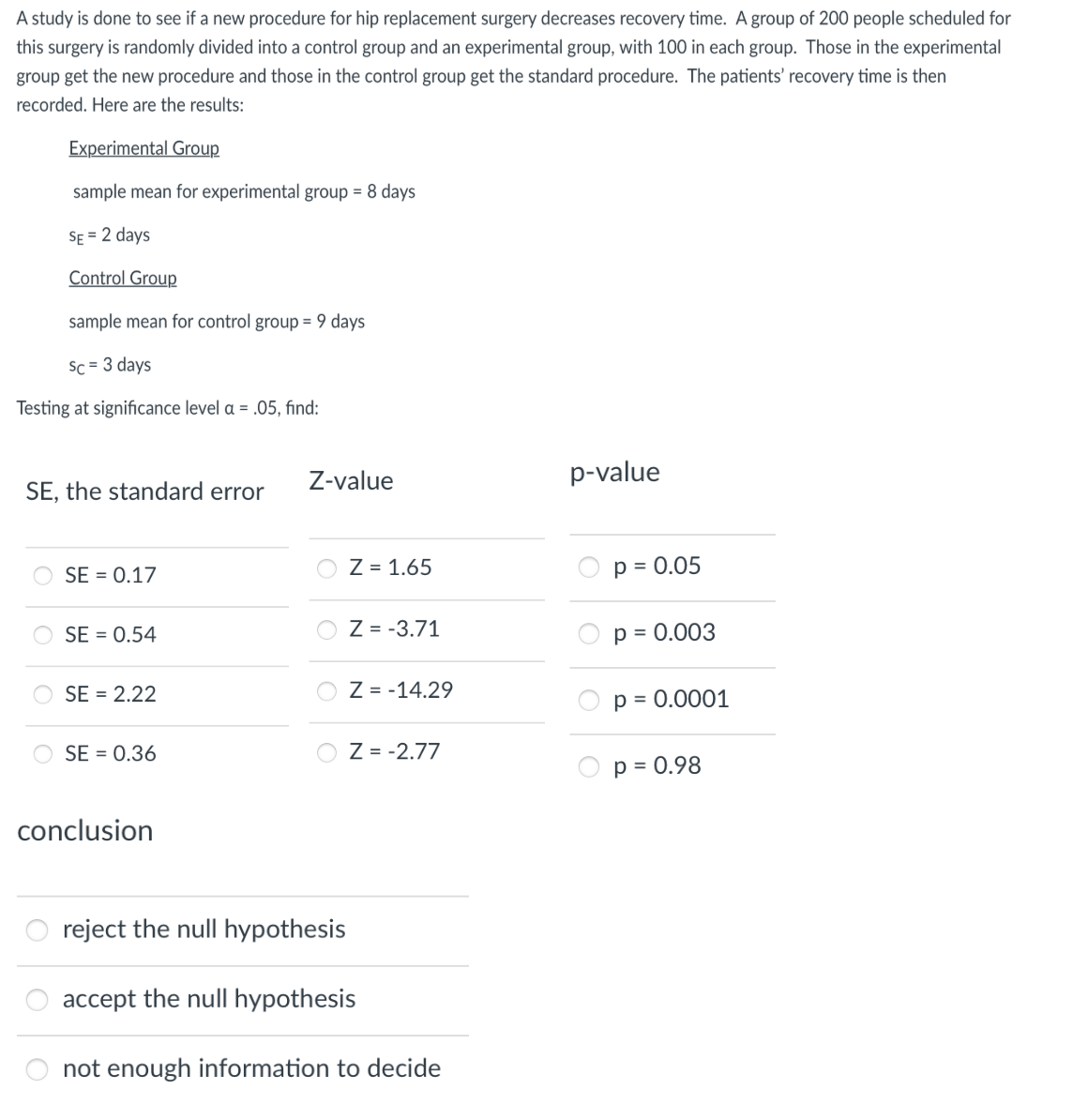Home / Expert Answers / Statistics and Probability / a-study-is-done-to-see-if-a-new-procedure-for-hip-replacement-surgery-decreases-recovery-time-a-gr-pa161

# (Solved): A study is done to see if a new procedure for hip replacement surgery decreases recovery time. A gr ...A study is done to see if a new procedure for hip replacement surgery decreases recovery time. A group of 200 people scheduled for this surgery is randomly divided into a control group and an experimental group, with 100 in each group. Those in the experimental group get the new procedure and those in the control group get the standard procedure. The patients' recovery time is then recorded. Here are the results: Experimental Group sample mean for experimental group days days Control Group sample mean for control group days days Testing at significance level , find: SE, the standard error Z-value -value conclusion reject the null hypothesis accept the null hypothesis not enough information to decide

We have an Answer from Expert

To find the standard error (SE), we need the sample size (n) and the standard deviation (s) of each group. In this case, the sample size for both groups is 100, and we are given the standard deviations: sE = 2 days (experimental group) and sC = 3 days (control group).
The standard error can be calculated as:

where nE is the sample size of the experimental group and nC is the sample size of the control group.

We have an Answer from Expert